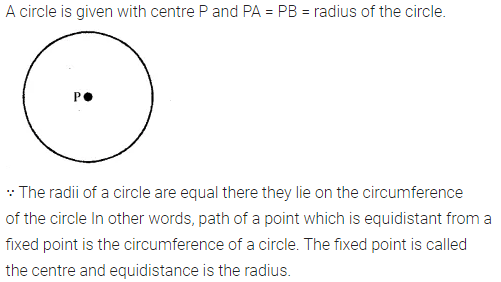# Selina Concise Mathematics Class 6 ICSE Solutions Chapter 23 Fundamental Concepts

## Selina Concise Mathematics Class 6 ICSE Solutions Chapter 23 Fundamental Concepts

Selina Publishers Concise Mathematics Class 6 ICSE Solutions Chapter 23 Fundamental Concepts

### Fundamental Concepts Exercise 23A – Selina Concise Mathematics Class 6 ICSE Solutions

Question 1.
State, true or false, if false, correct the statement.
(i) A dot has width but no length.
(ii) A ray has an infinite length only on one side of it.
(iii) A line segment PQ is written as $$\overleftrightarrow { PQ }$$ .
(iv) $$\overleftrightarrow { PQ }$$  represents a straight line.
(v) Three points are said to be collinear, if they lie in the same plane.
(vi) Three or more points all lying in the same line, are called collinear points.
Solution:Question 2.
Write how many lines can be drawn through :
(i) a given point?
(ii) two given fixed points?
(iii) three collinear points?
(iv) three non-collinear points?
Solution:Question 3.
The shaded region of the given figure shows a plane :
(a) Name :
(i) three collinear points.
(ii) three non-collinear points.
(iii) a pair of intersecting lines.
(b) State whether true or false :
(i) Line DE is contained in the given plane P.
(ii) Lines AB and DE intersect at point C.
(iii) Points D, B and C are collinear.
(iv) Points D, B and E are collinear.
Solution: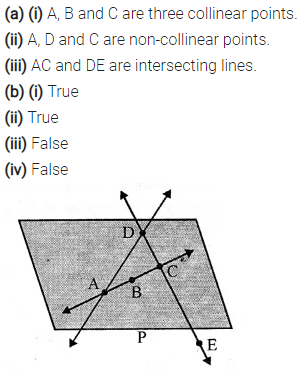Question 4.
Correct the statement, if it is wrong:
(i) A A ray can be extended infinitely on either side.
(ii) A ray has a definite length.
(iii) A line segment has a definite length.
(iv) A line has two end-points.
(v) A ray has only one end point.
Solution:Question 5.
State true-er false, if false give the correct statement :
(i) A line has a countable number of points in it.
(ii) Only one line can pass through a given point.
(iii) The intersection of two planes is a straight line
Solution: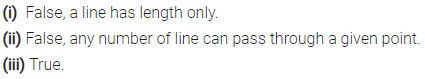Question 6.
State, whether the following pairs of lines or rays appear to be parallel or intersecting.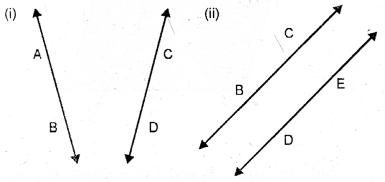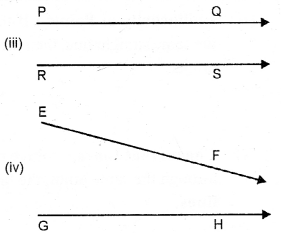Solution:Question 7.
Give two examples, from your surroundings, for each of the following:
(i) points
(ii) line segments
(iii) plane surfaces
(iv) curved surfaces.
Solution:Question 8.
Under what condition will two straight
lines, in the same plane, have :
(i) no point in common.
(ii) only one point in common.
(iii) an infinite number of points in common.
Solution: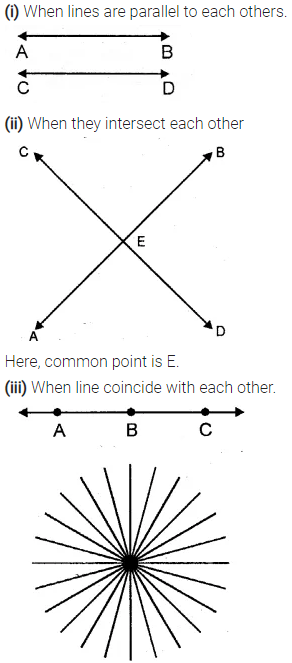Question 9.
Mark two points A and B on a page of your exercise book. Mark a third point P, such that :
(i) P lies between A and B; and the three points A, P and B are collinear.
(ii) P does not lie between A and B yet the three points are collinear.
(iii) the three points do not lie in a line.
Solution: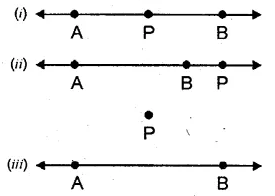Question 10.
Mark two points P and Q on a piece of paper. How many lines can you draw:
(i) passing through both the points P and Q?
(ii) passing through the point P ?
(iii) passing through the point Q ?
Solution: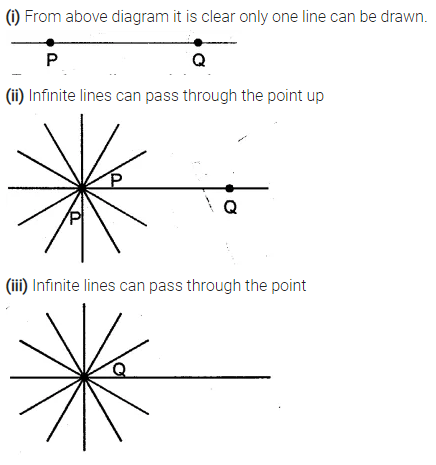Question 11.
The adjoining diagram shows a line AB. Draw diagram to represent:
(i) ray AB i.e. $$\overrightarrow { AB }$$(ii) $$\overrightarrow { BA }$$
(iii) line segment AB.
Solution:Question 12.
The adjoining diagram shows a ray AB. Draw diagrams to show :
(i) ray BA i.e. $$\overrightarrow { BA }$$
(ii) lineAB
(iii) line segment BA.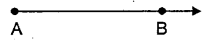Solution:Question 13.
The adjoining diagram shows a line segment AB. Draw diagrams to represent :
(i) ray AB i.e. $$\overrightarrow { AB }$$
(ii) line AB i.e. $$\overleftrightarrow { AB }$$
(iii) ray BA.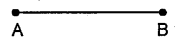Solution: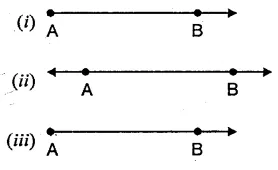Question 14.
Use a ruler and And whether the following points are collinear or not:
(i) D, A and C
(ii) A, B and C
(iii) A, B and E
(iv) B, C and E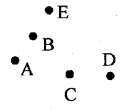Solution:Question 15.
(i) all pairs f parallel lines
(ii) all the lines which intersect EF.
(iii) lines whose point of intersection is G.Solution:### Fundamental Concepts Exercise 23B – Selina Concise Mathematics Class 6 ICSE Solutions

Question 1.
State, which of the following is a plane closed figure :Solution: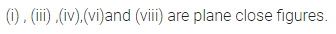Question 2.
Fill in the blanks :
(i) ….. is a three sided plane closed figure.
(ii) A square is a plane closed figure which is not bounded by …..
(iii) A rectangle is a …. sided plane ……
(iv) A rectangle has opposite sides ….. and adjacent sides ….. to each other.
(v) The sides of a square are ……. to each other and each angle is
(vi) For a line segment, a line making angle of ……… with it, is called perpendicular to it.
(vii) For a line segment, a line ……. it and making angle of ……. with it, is called ……. bisector of the line segment.
(viii) How many perpendiculars can be drawn to a line segment of length 6 cm ?
(ix) How many perpendicular bisectors can be drawn to a line segment of length 6 cm ?
(x) A perpendicular to a line segment will be its perpendicular bisector if it passes through the ……. of the given line segment.
Solution: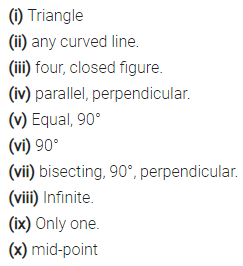Question 3.
State, which of the lines/line- segments are perpendicular to the line PQ :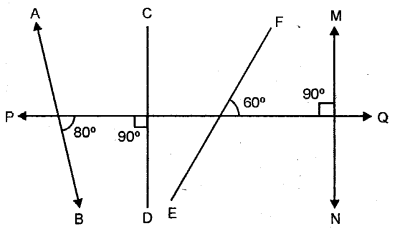Solution: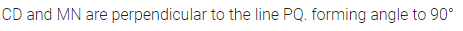Question 4.
Which of the following figures show two mutually perpendicular lines :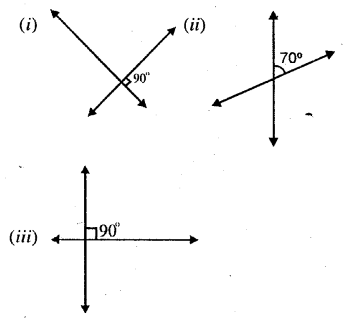Solution: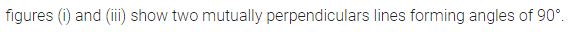Question 5.
For each figure given below name the line segment that is perpendicular bisector of the other :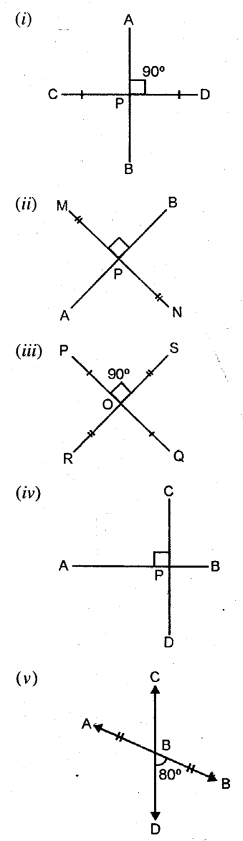Solution: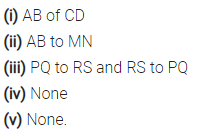Question 6.
Name three objects from your surroundings that contain perpendicular edges.
Solution: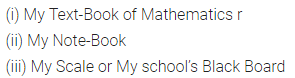Question 7.
Using the given figure, answer the following :
(i) Name the pairs of parallel lines.
(ii) Name the pairs of mutually perpendicular lines.
(iii) Is the line p parallel to the line l ?
(iv) Is the line q perpendicular to the line m ?Solution:Question 8.
Place a scale (ruler) on a sheet of paper and hold it firmly with one hand. Now draw two line segments AB and CD along the longer edges of the scale. State whether segment AB is parallel to or perpendicular to segment CD.
Solution: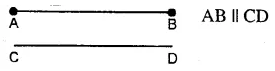Question 9.
(i) How many pairs of its edges are parallel to each other ?
(ii) How many pairs of its edges are perpendicular to each other ?
Solution: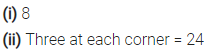Question 10.
Give two examples from your surroundings for each of the following :
(i) intersecting lines
(ii) parallel lines
(iii) perpendicular lines.
Solution:Question 11.
State true or false; if false, give the correct statement :
(i) The maximum number of lines through three collinear points is three.
(ii) The maximum number of lines through three non-collinear points is three.
(iii) Two parallel lines always lie in the same plane.
(iv) Concurrent lines always meet at the same point.
(v) A surface can be plane or curved.
(vi) There are an infinite number of points in a line segment of length 10 cm.
(vii) There are an infinite number of points in a line.
(viii) A plane has an infinite number of lines.
(ix) A plane has an infinite number of points.
Solution: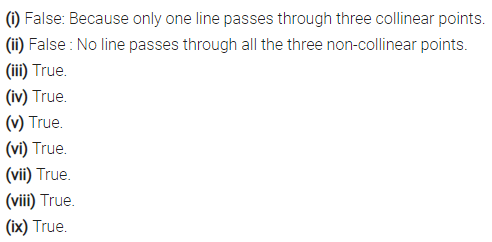### Fundamental Concepts Revision Exercise – Selina Concise Mathematics Class 6 ICSE Solutions

Question 1.
Mark a point O on a piece of paper. Using a sharp pencil and a ruler, draw a line through the point O. Then draw one more line through the point O. How many lines can you draw through O?
Write a statement regarding your observations.
Solution: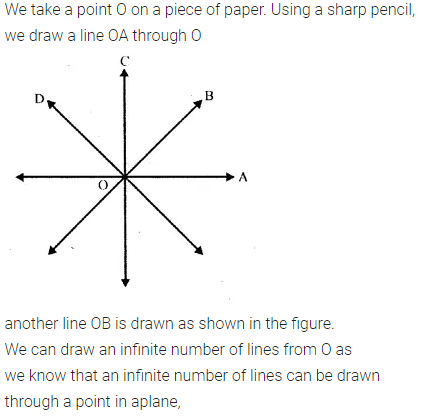Question 2.
Mark two points A and B on a plain sheet “ of paper. Using a sharp pencil and a ruler, draw a line through points A and B. Try to draw one more line through A and B. What do you observe ?
Write a statement regarding your observations.
Solution: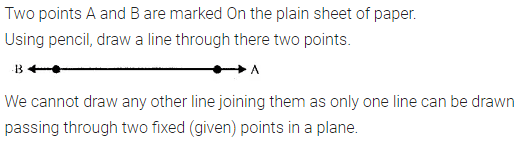Question 3.
Draw two straight lines on a sheet of paper so that the lines drawn :
(i) intersect each other.
(ii) do not intersect.
(iii) appear to intersect when extended.
Solution: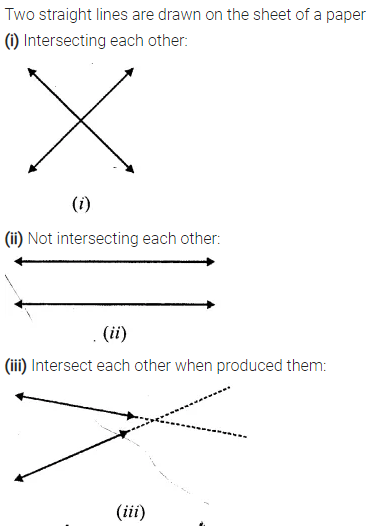Question 4.
Draw a figure to show that :
(i) points A, B and C arc collinear.
(ii) lines AB, CD and EF are concurrent.
Solution:Question 5.
In your note-book, draw two rays with the same initial point O and going in opposite directions. Write the special name for the final figure obtained.
Solution: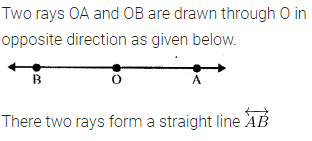Question 6.
In each figure, given below, write the number of line segments used :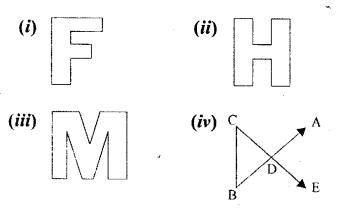Solution: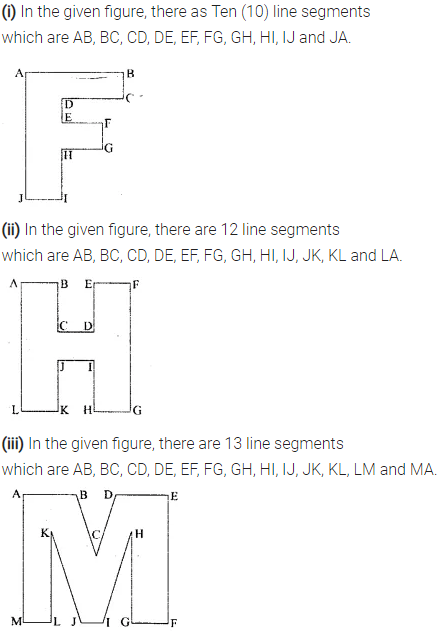Question 7.
In a circle, point P is its centre and PA = PB = radius of the circle. Where do points A and B lie ?
Solution:Question 8.
Draw a four-sided closed figure, in which:
(i) all the sides are equal and each angle is 90°.
(ii) opposite sides are equal and each angle is 90°.
Write the special name of each figure drawn.
Solution: